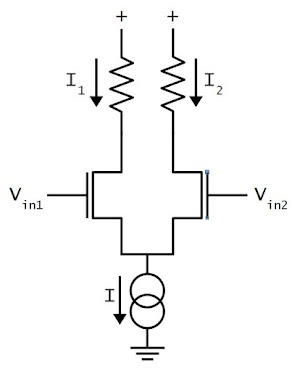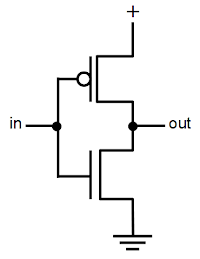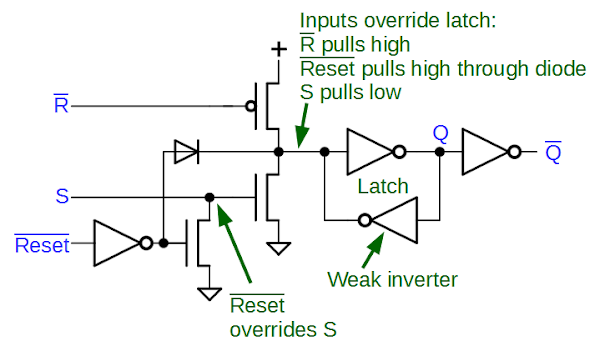Browse over 10,000 Electronics Projects

# Reverse engineering the popular 555 timer chip (CMOS version)### IC component: The differential pair

The second important circuit to understand is the differential pair, the most common two-transistor subcircuit used in analog ICs.
You may have wondered how a comparator compares two voltages, or an op amp subtracts two voltages. This is the job of the differential pair.Schematic of a simple differential pair circuit. The current sink sends a fixed current I through the differential pair. If the two inputs are equal, the current is split equally between the two branches. Otherwise, the branch with the higher input voltage gets most of the current.

The schematic above shows a simple differential pair. The current source at the bottom sinks a fixed current I, which is split between the two input transistors. If the input voltages are equal, the current will be split equally into the two branches (I1 and I2). If one of the input voltages is a bit higher than the other, the corresponding transistor will conduct more current, so one branch gets more current and the other branch gets less.
A small input difference is enough to direct most of the current into the “winning” branch, flipping the comparator on or off.

Rather than resistors, the chip uses a current mirror on the two branches.
This acts as an active load and increases the amplification.

## Inverters and the flip flop

Although the 555 is an analog circuit, it contains a digital flip flop to remember its state.
The flip flop is built out of inverters, simple logic circuits that turn a 1 into a 0 and vice versa. The 555 uses standard CMOS inverters, as shown below.Structure of a CMOS inverter: a PMOS transistor at top and a NMOS transistor at bottom.

The inverter is built from two transistors.
If the input is 0 (i.e. low), the PMOS transistor on top turns on, connecting
the positive supply to the output, producing a 1.
If the input is 1 (high), the NMOS transistor on the bottom turns on, connecting ground to the output, producing a 0.
The magical part of CMOS is that the circuit uses almost no power. Current doesn’t flow through the gate (because of the insulating oxide layer), so the only power usage is a tiny pulse when the output changes state, to charge or discharge the wire’s capacitance.

The diagram below shows the circuit for the flip flop.
Two inverters are connected in a loop to form a latch. If the top inverter outputs 1, the bottom outputs 0, forming a stable cycle.
If the top inverter outputs 0, the bottom outputs 1, again forming a stable cycle.Circuit diagram of the flip flop in the LMC555 CMOS timer chip.

To change the value stored in the flip flop, the new value is simply forced into the latch, overriding the existing value with brute force.
To make this work, the bottom inverter is “weak”, using low-current transistors.
This allows the set or reset inputs to overpower the weak inverter and
the latch will immediately flip into the proper state
The R (reset) and S (set) inputs come from the comparators and pull the latch input high or low through the transistors.
Reset comes from the input pin
and
pulls the latch input high through a diode; the Reset inverter’s output current is controlled by a current mirror.
Reset will pull S low, blocking the action of a contradictory S input.

Pages: 1 2 3 4 5 6

Top